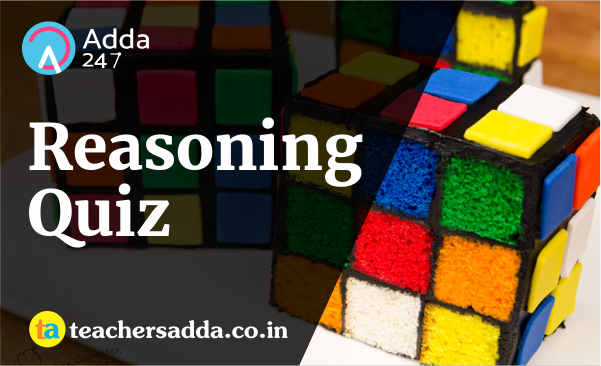The reasoning is the most scoring section in any competitive exam. It also develops or nourishes our critical thinking skills as compared to other subjects. In DSSSB,KVS Exam, As a student to score well in this part, you have to start your preparation now because there is negative marking as well. The more you have prepared, the better you score.

Directions (1-2): A series is given with one term missing. Choose the correct alternative from the given ones that will complete the series.

Q1. RP, DM, SQ, CL, TR, ?
(a) AJ
(b) US
(c) DM
(d) BK

Q2. –1, 1, 4, 9, 16, ?
(a) 24
(b) 27
(c) 26
(d) 36

Directions (3): In the following question, two statements are given each followed by two conclusions I and II. You have to consider the statements to be true even if they seem to be at variance from commonly known facts. You have to decide which of the given conclusions, if any, follows from the given statements.

Q3. Statements:
I. Some notes are coins.
II. No coin is a card.

Conclusion:
I. All cards can be notes.
II. Some notes are neither coins nor cards
.

(a) Conclusion I follows
(b) Conclusion II follows
(c) Neither I nor II follows
(d) Both I and II follows

Q4. If 27th December 2009 was a Thursday, then what day of the week was it on 1st March 2010?
(a) Thursday
(b) Friday
(c) Sunday
(d) Monday

Q5. Arrange the given words in the sequence in which they occur in the dictionary.
i. Obstacle
ii. Obscure
iii. Obsession
iv. Obstruct

(a) iii, iv, i, ii
(b) i, iv, iii, ii
(c) ii, iii, i, iv
(d) iv, iii, ii, i

Q6. In a certain code language, “REMINDER” is written as “SFNJMCDQ”. How is “STANDARD” written in that code language?
(a) TUBOEQZE
(b) CZQETUBO
(c) TUBOCZQC
(d) TUBOEBSEQ8. If “A” denotes “added to”, “B” denotes “divided by”, “C” denotes “multiplied by” and “D” denotes “subtracted from”, then 116 B 29 C 6 A 24 D 45 = ?
(a) 4
(b) 3
(c) 5
(d) 6

Q9. Which set of letters when sequentially placed at the gaps in the given letter series shall complete it?
_ r _ r _ t _ r _ r _ t

(a) tpprpp
(b) ttprrp
(c) tpptpp
(d) ttpprp

Q10. A man is facing towards the east. He turns 270 degrees clockwise and then takes a right turn. Finally, he turns 90 degrees anticlockwise. Which direction is he facing now?
(a) West
(b) South
(c) North
(d) East